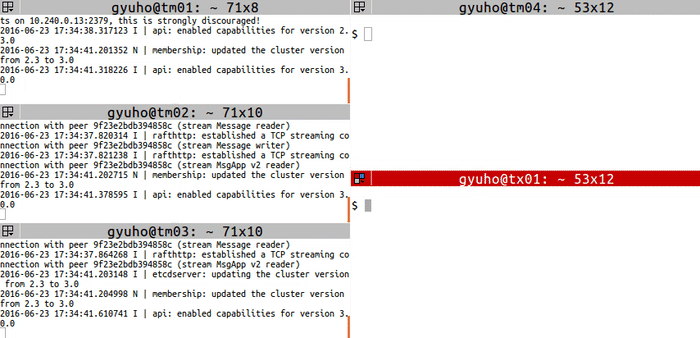# How to Add and Remove Members

Guide to dealing with membership in etcd cluster

`member` to add,remove,update membership:``````# For each machine
TOKEN=my-etcd-token-1
CLUSTER_STATE=new
NAME_1=etcd-node-1
NAME_2=etcd-node-2
NAME_3=etcd-node-3
HOST_1=10.240.0.13
HOST_2=10.240.0.14
HOST_3=10.240.0.15
CLUSTER=\${NAME_1}=http://\${HOST_1}:2380,\${NAME_2}=http://\${HOST_2}:2380,\${NAME_3}=http://\${HOST_3}:2380

# For node 1
THIS_NAME=\${NAME_1}
THIS_IP=\${HOST_1}
etcd --data-dir=data.etcd --name \${THIS_NAME} \
--listen-peer-urls http://\${THIS_IP}:2380 \
--listen-client-urls http://\${THIS_IP}:2379 \
--initial-cluster \${CLUSTER} \
--initial-cluster-state \${CLUSTER_STATE} \
--initial-cluster-token \${TOKEN}

# For node 2
THIS_NAME=\${NAME_2}
THIS_IP=\${HOST_2}
etcd --data-dir=data.etcd --name \${THIS_NAME} \
--listen-peer-urls http://\${THIS_IP}:2380 \
--listen-client-urls http://\${THIS_IP}:2379 \
--initial-cluster \${CLUSTER} \
--initial-cluster-state \${CLUSTER_STATE} \
--initial-cluster-token \${TOKEN}

# For node 3
THIS_NAME=\${NAME_3}
THIS_IP=\${HOST_3}
etcd --data-dir=data.etcd --name \${THIS_NAME} \
--listen-peer-urls http://\${THIS_IP}:2380 \
--listen-client-urls http://\${THIS_IP}:2379 \
--initial-cluster \${CLUSTER} \
--initial-cluster-state \${CLUSTER_STATE} \
--initial-cluster-token \${TOKEN}
``````

Then replace a member with `member remove` and `member add` commands:

``````# get member ID
export ETCDCTL_API=3
HOST_1=10.240.0.13
HOST_2=10.240.0.14
HOST_3=10.240.0.15
etcdctl --endpoints=\${HOST_1}:2379,\${HOST_2}:2379,\${HOST_3}:2379 member list

# remove the member
MEMBER_ID=278c654c9a6dfd3b
etcdctl --endpoints=\${HOST_1}:2379,\${HOST_2}:2379,\${HOST_3}:2379 \
member remove \${MEMBER_ID}

# add a new member (node 4)
export ETCDCTL_API=3
NAME_1=etcd-node-1
NAME_2=etcd-node-2
NAME_4=etcd-node-4
HOST_1=10.240.0.13
HOST_2=10.240.0.14
HOST_4=10.240.0.16 # new member
etcdctl --endpoints=\${HOST_1}:2379,\${HOST_2}:2379 \
member add \${NAME_4} \
--peer-urls=http://\${HOST_4}:2380
``````

Next, start the new member with `--initial-cluster-state existing` flag:

``````# [WARNING] If the new member starts from the same disk space,
# make sure to remove the data directory of the old member
#
TOKEN=my-etcd-token-1
CLUSTER_STATE=existing
NAME_1=etcd-node-1
NAME_2=etcd-node-2
NAME_4=etcd-node-4
HOST_1=10.240.0.13
HOST_2=10.240.0.14
HOST_4=10.240.0.16 # new member
CLUSTER=\${NAME_1}=http://\${HOST_1}:2380,\${NAME_2}=http://\${HOST_2}:2380,\${NAME_4}=http://\${HOST_4}:2380

THIS_NAME=\${NAME_4}
THIS_IP=\${HOST_4}
etcd --data-dir=data.etcd --name \${THIS_NAME} \